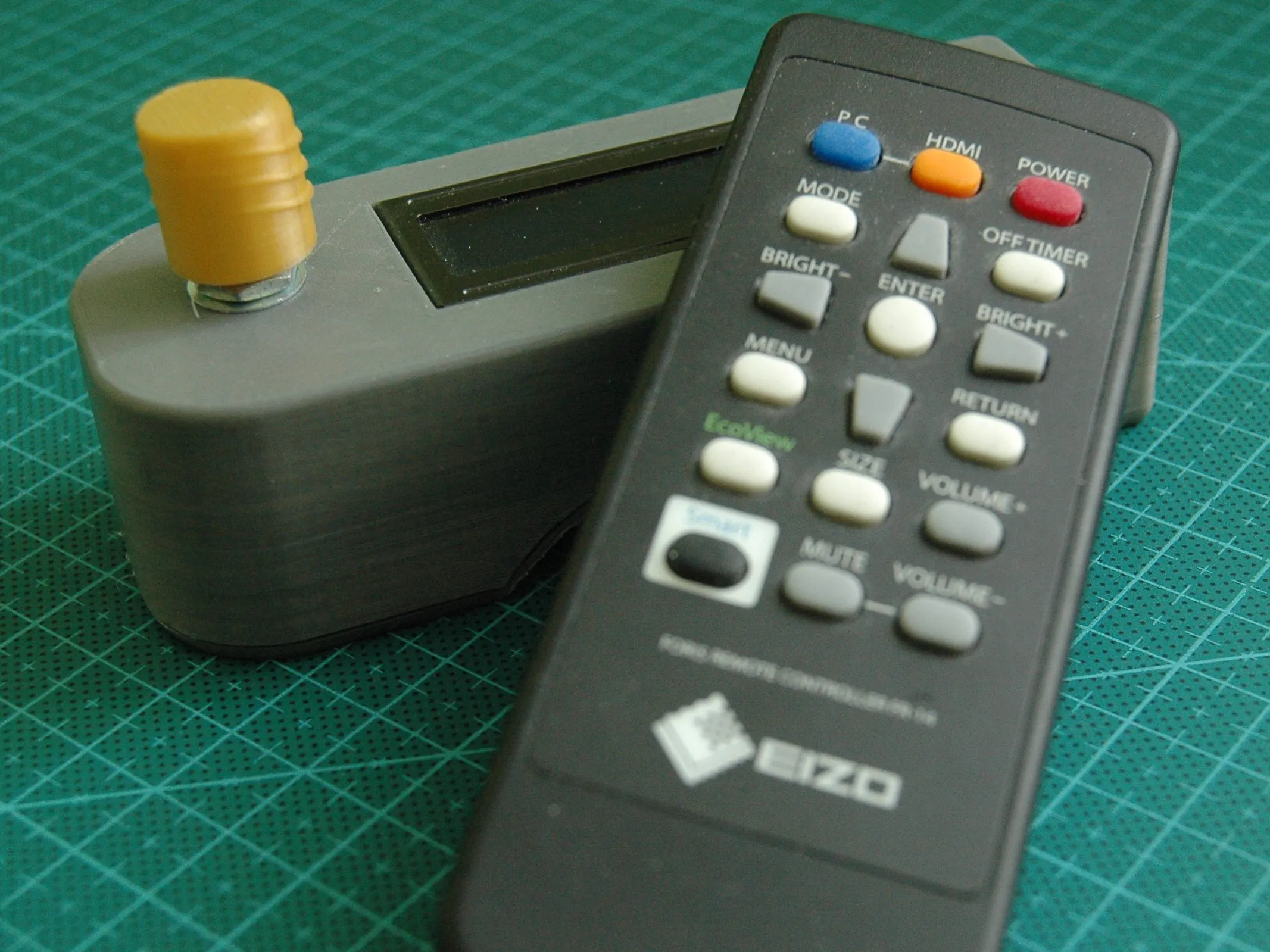Project showcaseNow you don't have to remember any of your passwords, and they are still safe and ready to use!

• 10,753 views
• 36 respects

## Necessary tools and machines3D Printer (generic)

## Apps and online services

Hi everyone!

The device looks like this:

It doesn't have any power source inside, which is not necessary, because it only works in combination with the computer. In this project I have used the Arduino Pro Micro because of the ATmega32U4 chip that already has built in USB.

To connect the device to the computer we need a standard smartphone charging cable (USB micro).

Once connected you have to type in only one password to get access to the other ones. You can change it in the sketch. The password is the rotation clicks of the encoder in the right direction the right number of times. It is difficult to understand, just watch the video to see what I am writing about)

Use the encoder to navigate through the menu and press the encoder to enter password into the computer.

Here is the video where I am building it. Watch it please and leave a like!

This really supports me.

For the components check out my website: https://www.allmakers.net/

I am open for any kind of questions and suggestions. Feel free to comment!

## Code

##### The codeC/C++
```#include <Keyboard.h>                                 //
#include <LiquidCrystal_I2C.h>                        //
#include <EEPROM.h>
#define pinA 5                                        //
#define pinB 6                                        //
#define btnPin 4                                      //

byte aState;                                          //
byte bState;                                          //
byte aLastState;                                      //
int dir;                                              //-1 -  . ., 1 -  . .
byte attempt = 0;                                     //-
int pos = 0;                                          //
byte typed = 0;                                       //
bool accepted = false;                                //

String code = "101";                                  //  : 101 -- 110011
int codeLen = 0;                                      //
String enter = "";                                    //

int a = 0;                                                //
int len = 0;                                              //
int counter;

byte address = 0;
long currentTime;

{"EPN", ""},
{"Mail", ""},
{"Asus", ";"},
{"GMX", ""}
};

LiquidCrystal_I2C lcd(0x3B, 16, 2);                   //

int lcdClear(byte x) {                                //
if (x == 2) {                                       //2 clear all, 0 clear first line, 1 clear second line
for (int i = 0; i < 2; ++i) {
lcd.setCursor(0, i);
lcd.print("                ");
}
}
else {
lcd.setCursor(0, x);
lcd.print("                ");
}
}

void setup() {
pinMode(pinA, INPUT);                               //  -
pinMode(pinB, INPUT);                               //  -
pinMode(btnPin, INPUT);                             // -
Serial.begin(9600);                                 //
Keyboard.begin();                                   //
aLastState = digitalRead(pinA);                     //  .
lcd.init();                                         //
lcd.backlight();                                    //
lcd.setCursor(0, 0);
lcd.setCursor(1, 1);
lcd.print("Version 1.6 PM");
delay(3000);
lcdClear(2);
while (passwords[a] != NULL) {
a++;
len++;
}
//Serial.println(len);
}

void getDirection() {
aState = digitalRead(pinA);                         //
if (aState != aLastState) {                         // ...
if (aState == digitalRead(pinB)) {                           //  ...
//Serial.println("CCW 0");                        //
dir = -1;                                       //  . .
}
else {
//Serial.println("CW 1");                         //
dir = 1;                                        //  . .
}
}
aLastState = aState;                                //  .
}

void convert() {                                      //   ,
for (int k = 0; k < codeLen; k++) {
enter.remove(k, 1);
}
}

void check() {
if (enter == code) {
accepted = true;                                //
lcdClear(2);
lcd.setCursor(4, 0);
lcd.print("Welcome!");
delay(2000);
lcdClear(2);
enter = "";
typed = 0;
lcd.setCursor(0, 0);
lcd.print(">");
if (len > 1) {
lcd.setCursor(1, 1);
}
}
else {
attempt += 1;                                    //-
if (attempt == 3) {
lcdClear(2);
lcd.setCursor(0, 0);
lcd.setCursor(0, 1);
lcd.print("Wait 1 minute!");
currentTime = millis();
while (millis() - currentTime < 1000 * 60) {
currentTime = millis();
}
attempt = 0;
lcdClear(2);
typed = 0;
}
else {
lcd.setCursor(7, 0);
lcd.print("         ");
lcd.setCursor(15, 1);
lcd.print(3 - attempt);
lcd.setCursor(7, 0);
}
accepted = false;                                //
enter = "";
}
}

void loop() {
if (accepted == false) {
lcdClear(2);
lcd.setCursor(0,0);
lcd.print("      WAIT      ");
delay(1000 * 30);
lcdClear(2);
delay(1000);
}
getDirection();                                 //
if (typed == 0) {
lcd.setCursor(0, 0);
lcd.print("Unlock:");
lcd.setCursor(0, 1);
lcd.print("Attempts left: 3");
typed = 1;
lcd.setCursor(7, 0);
}
if (dir != 0) {
if (dir == -1) {
enter = enter + "0";
}
else {
enter = enter + "1";
}
codeLen = enter.length();                         //
if (codeLen % 2 == 0) {
lcd.print("*");
}
dir = 0;
}
if (digitalRead(btnPin) == 0) {                       //  ...
delay(300);
convert();
check();
}
}

else {
getDirection();
if (dir == 1 || dir == -1) {
counter++;
if (counter % 2 == 0) {
counter = 0;
if (dir == 1) {
if (pos + 1 < len) {
if (pos % 2 == 0) {
pos++;
lcd.setCursor(0, 0);
lcd.print(" ");
lcd.setCursor(0, 1);
lcd.print(">");
}
else {
pos++;
lcdClear(2);
lcd.setCursor(0, 0);
lcd.print(">");
if (pos + 1 <= len) {
lcd.setCursor(1, 1);
}
}
}
}
else {
if (pos - 1 >= 0) {
if (pos % 2 == 0) {
pos--;
lcdClear(2);
lcd.setCursor(0, 1);
lcd.print(">");
lcd.setCursor(1, 0);
}
else {
pos--;
lcd.setCursor(0, 1);
lcd.print(" ");
lcd.setCursor(0, 0);
lcd.print(">");
}
}
}
}
dir = 0;
}
if (digitalRead(btnPin) == 0) {
delay(300);
}
}
}
```

## Schematics#### Automated NERF Gun Shooting Gallery

Project showcase by Keegan Neave

• 2,819 views
• 1 comment
• 12 respects

#### Arduino and Android Based Password Protected Door Lock

Project tutorial by Md. Khairul Alam

• 12,094 views
• 46 respects

#### High Precision Scale with Arduino

Project showcase by mPelectronic

• 8,897 views
• 37 respects

#### Security System Using Password by Arduino & Python

Project in progress by muhammed amar slik

• 3,532 views
• 13 respects

#### Automated Garden

Project tutorial by Thomas sxt

• 21,620 views